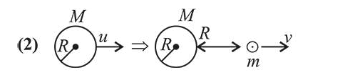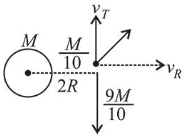# A satellite of mass m is launched vertically upwards with an initial speed u from the surface of the earth.

Question:

A satellite of mass $m$ is launched vertically upwards with an initial speed $u$ from the surface of the earth. After it reaches height $R$ ( $R=$ radius of the earth $)$, it

ejects a rocket of mass $\frac{m}{10}$ so that subsequently the

satellite moves in a circular orbit. The kinetic energy

of the rocket is ( $G$ is the gravitational constant; $M$ is the mass of the earth):

1. (1) $\frac{m}{20}\left(u^{2}+\frac{113}{200} \frac{G M}{R}\right)$

2. (2) $5 m\left(u^{2}-\frac{119}{200} \frac{G M}{R}\right)$

3. (3) $\frac{3 m}{8}\left(u+\sqrt{\frac{5 G M}{6 R}}\right)^{2}$

4. (4) $\frac{m}{20}\left(u-\sqrt{\frac{2 G M}{3 R}}\right)^{2}$

Correct Option: , 2

Solution:$\frac{1}{2} m u^{2}+\frac{-G M m}{R}=\frac{1}{2} m v^{2}+\frac{-G M m}{2 R}$

$\Rightarrow \frac{1}{2} m\left(v^{2}-u^{2}\right)=\frac{-G M m}{2 R}$

$\Rightarrow V=\sqrt{V=u^{2}-\frac{G M}{R}}$                ...(1)

$v_{0}=\sqrt{\frac{G M}{2 R}}$        $\therefore v_{\text {rad }}=\frac{m \times v}{\left(\frac{m}{10}\right)}=10 v$

Ejecting a rocket of mass $\frac{m}{10}$

$\therefore \frac{9 m}{10} \times \sqrt{\frac{G M}{2 R}}=\frac{m}{10} \times v_{\tau} \Rightarrow V_{\tau}^{2}=81 \frac{G M}{2 R}$

Kinetic energy of rocket,

$\mathrm{KE}_{\text {rocket }}=\frac{1}{2} \frac{M}{10}\left(V_{T}^{2}+V_{r}^{2}\right)=\frac{1}{2} \times \frac{m}{10} \times\left(\left(u^{2}-\frac{G M}{R}\right) 100+81 \frac{G M}{R}\right)$

$=\frac{m}{20} \times 100\left(u^{2}-\frac{G M}{R}+\frac{81}{200} \frac{G M}{R}\right)$

$=5 m\left(u^{2}-\frac{119}{200} \frac{G M}{R}\right)$